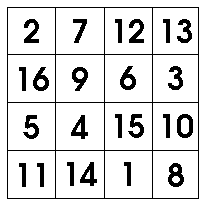# Magic Numbers of Magic Squares

### How To Work Out What Each Row Of A Magic Square Should Add To

By

A magic square is a grid containing the numbers 1, 2, 3, and so on, where each row, column and diagonal add up to the same number. An example is shown below, you will see that each row, column and diagonal add up to 34. This number 34 is the "magic number" of the magic square.Finding magic squares or solving magic square puzzles is much easier if you know the magic number. The good news is, once you know the size of the magic square you want, you can calculate the magic number without too much trouble. This is because of an amazing fact :

• The magic number depends only on the size of the square grid, not on how the numbers are arranged within it

Why is this? Suppose you add up each row of a magic square. Each row will add up to the magic number. So if you add all the cells together, you have added up this magic number as many times as there are rows in the grid. Let's note this fact:

• The sum of all the cells in the grid is the magic number times the number of rows

But there's another way to add all the cells together, since each number 1, 2, 3 and so on appears exactly once. The biggest number in the grid is the number of rows times the number of columns. Therefore,

• The sum of all the cells in the grid is the sum of all the numbers from 1 up to the number of rows times the number of columns.

A magic square has the same number of rows as columns, so the magic number depends only on the number of rows in the magic square. The following steps help you work it out :

• Take the number of rows, and multiply this by itself
• multiply again by the number of rows.
• divide by two

For example, for the four by four square above (or for any four by four magic square), the magic number can be worked out as :

• four times four is 16,
• add one, gives 17
• multiply again by four, gives 68
• divide by two, gives 34!

To save you some calculations, I've given below the magic numbers of a few different sizes of magic square :

• for a 3 by 3 square, the magic number is 15.
• for a 4 by 4 square, the magic number is 34.
• for a 5 by 5 square, the magic number is 65.
• for a 6 by 6 square, the magic number is 111.
• for a 7 by 7 square, the magic number is 175.
• for a 8 by 8 square, the magic number is 260.
• for a 9 by 9 square, the magic number is 369.
• for a 10 by 10 square, the magic number is 505.

Yours, Dr Mike!

If you like what you've just read, sign up for this site's free newsletters:
• Monday Morning Math: A weekly email of fascinating math facts - how math works in everyday life.
• New Game Alerts: Be first to know when I upload new games.
Get these free now! Enter Your Email: